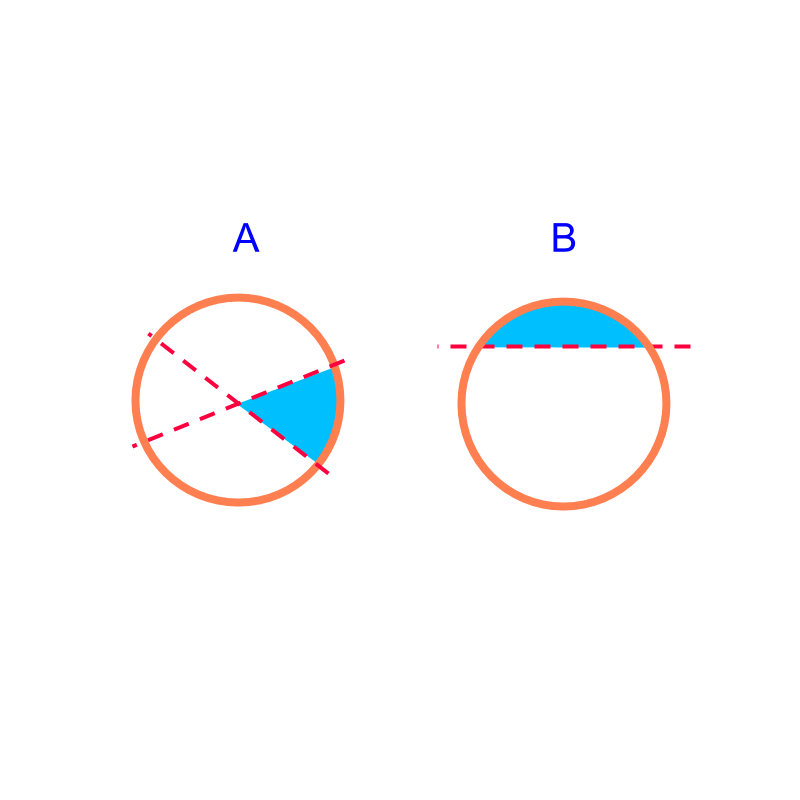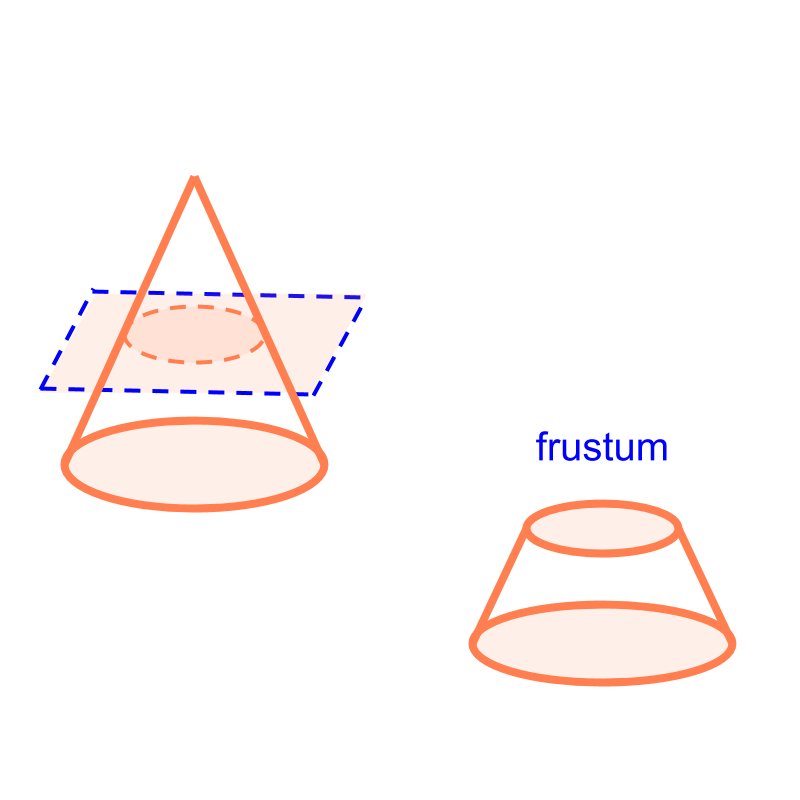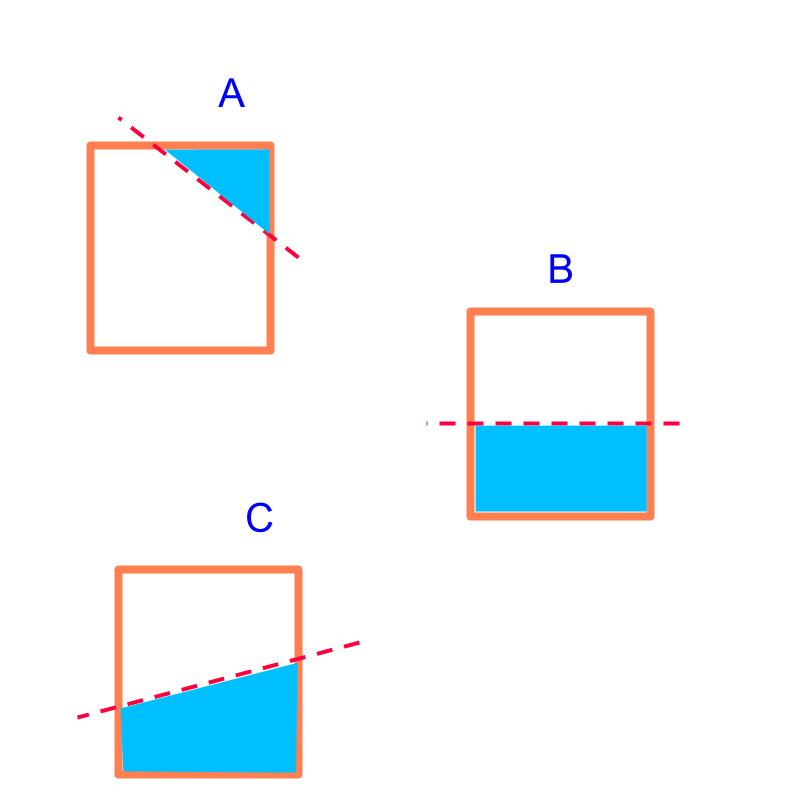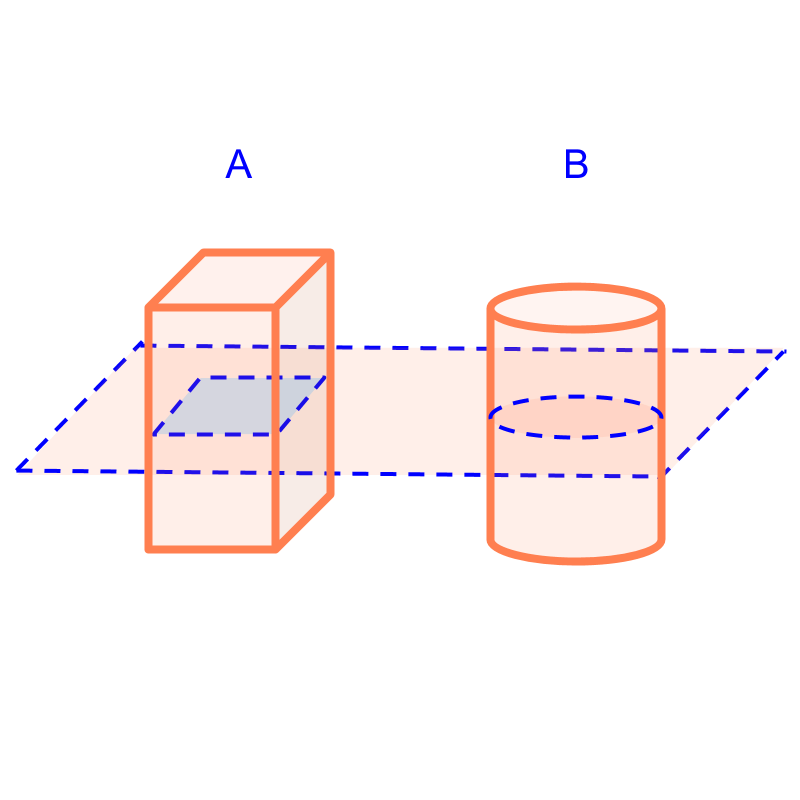maths > mensuration-high

Understanding Part Shapes

what you'll learn...

Overview

•  squares,

•  rectangles,

•  triangles,

•  polygons, and

•  circles These are some of the standard shapes.

Some part-shapes can be derived from these standard shapes. One of the method is to cut the shapes with straight lines and part of the standard shape is analyzed for perimeter or area.

The following are studied•  the perimeter and area of sectors and segments.•  the surface area and volume of frustum.

slicing rectangleConsider slicing rectangle as shown in the figure. The possible shapes formed in slicing a rectangle with a straight line are
triangle
rectangle

Part shapes are illustrated in the figure.

It is noted that for polygons, the part-shape will be another polygon.

slicing circleWhen a circle is sliced with a line, the shapes formed are "sector and segment", as shown in the figure.

Sector and Segment are studied in Geometry. In mensuration, the interest is in finding the perimeter and area of the part-shapes. In particular, the perimeter and area of sectors and segments are studied.

solids•  cubes,

•  cuboids,

•  cylinders,

•  cones,

•  prisms,

•  pyramids, and

•  spheres These are some of the standard solids.

Some part-solids can be derived from these standard shapes. One of the method is to cut the shapes with a plane and part of the standard shape is analyzed for surface area or volume.

Consider slicing a cuboid and a cylinder with a plane as shown in the figure. For this course, we restrict ourselves to planes parallel to one of the faces. The part-solids are formed from this are a cuboid and a cylinder.

slicing coneWhen a cone is sliced with a plane, the part-solid formed are shown in the figure. The two part-solids of cone are a cone and a frustum

summary

The part-shaped formed by planes, that are not parallel to a face of the solid, are outside the scope of this course. In mensuration, the interest is in finding the surface area and volume of the part-shapes. In particular, the following are studied•  the perimeter and area of sectors and segments.•  the surface area and volume of frustum.

Outline